Next: 15. The Second Derivative Up: 14. The Inverse Function Previous: 14.5 Inverse Function Theorems   Index

# 14.6 Some Derivative Calculations

14.46   Example (Derivative of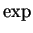.) We know that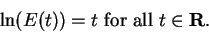If we differentiate both sides of this equation, we get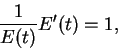i.e.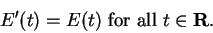14.47   Example (Derivative of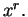) Let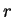be any real number and let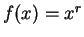for all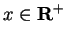. Then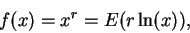so by the chain rule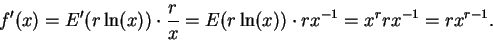(Here I have used the result of exercise 14.37.) Thus the formula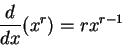which we have known for quite a while for rational exponents, is actually valid for all real exponents.

14.48   Exercise (Derivative of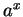.) Let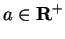. Show that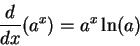for all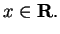14.49   Example (Derivative of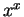.)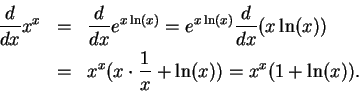Hence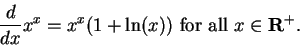14.50   Example (Derivative of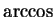.) Let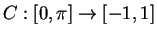be defined by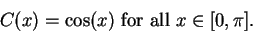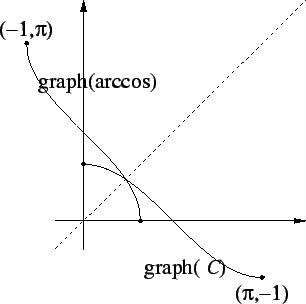We have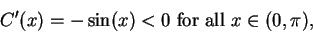so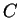has an inverse function which is denoted by. By the inverse function theoremis differentiable on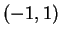. and we have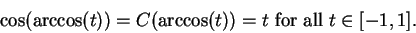By the chain rule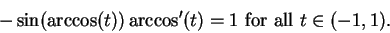Now since the sine function is positive on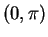we get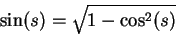for all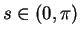, so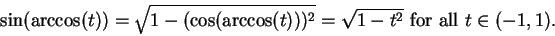Thus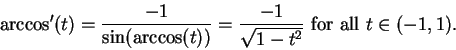14.51   Exercise (Derivative of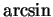.) Let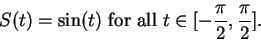Show that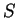has an inverse function that is differentiable on the interior of its domain. This inverse functions is called. Describe the domain of, sketch the graphs ofand of, and show that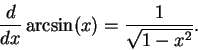14.52   Example (Derivative of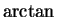.) Let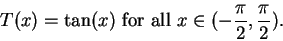Then T is continuous, and the image of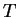is unbounded both above and below, so image() = R. Also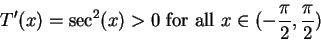sohas an inverse function, which we denote by.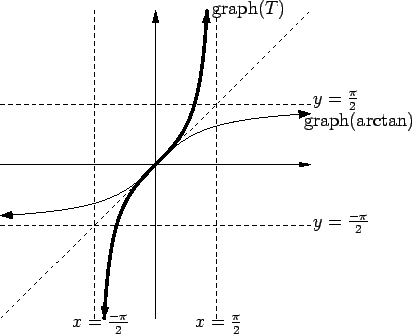For all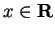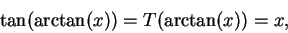so by the chain rule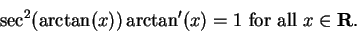Now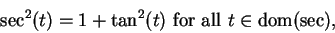so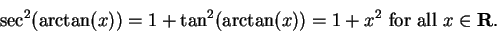Thus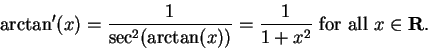14.53   Exercise (Derivative of arccot.) Let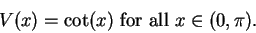Show that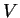has an inverse function arccot, and that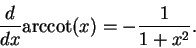What is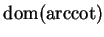? Sketch the graphs ofand of arccot.

Remark The first person to give a name to the inverse trigonometric functions was Daniel Bernoulli (1700-1792) who used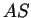forin 1729. Other early notations included arc(cos. =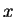) and ang(cos. =)[15, page 175]. Many calculators and some calculus books use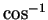to denote arccos. (If you use your calculator to find inverse trigonometric functions, make sure that you set the degree-radian-grad mode to radians.)

14.54   Exercise. A Calculate the derivatives of the following functions, and simplify your answers (Here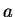is a constant.)
a)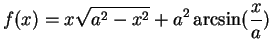.
b)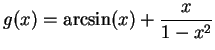.
c)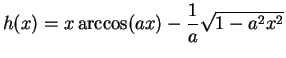.
d)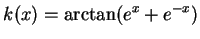.
e)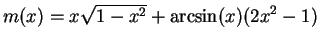.
f)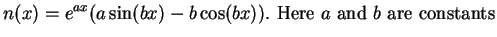.
g)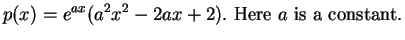.

14.55   Exercise. A Let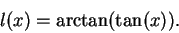Calculate the derivative of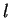. What is the domain of this function? Sketch the graph of.

14.56   Exercise (Hyperbolic functions.) We define functions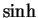and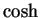on R by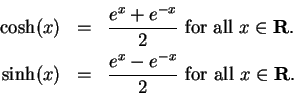These functions are called the hyperbolic sine and the hyperbolic cosine respectively. Show that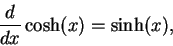and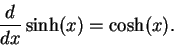Calculate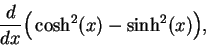and simplify your answer as much as you can. What conclusion can you draw from your answer? Sketch the graphs ofandon one set of coordinate axes.Next: 15. The Second Derivative Up: 14. The Inverse Function Previous: 14.5 Inverse Function Theorems   Index
Ray Mayer 2007-09-07